# Find the rule which gives the number of matchsticks required to make the following matchstick patterns. Use a variable to write the rule.(a) A pattern of letter $T$ as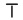(b) A pattern of letter $\mathrm{Z}$ as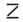(c) A pattern of letter $\mathrm{U}$ as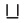(d) A pattern of letter $\mathrm{V}$ as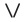(e) A pattern of letter $\mathrm{E}$ as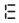(f) A pattern of letter $S$ as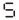(g) A pattern of letter A as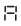"

To do:

We have to find the rule which gives the number of matchsticks required to make the given matchstick patterns.

Solution:

(a) A pattern of letter $T$ as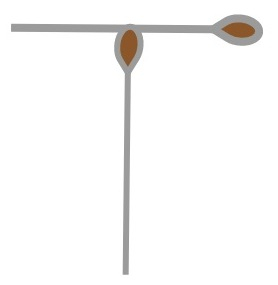From the figure, we observe that two matchsticks are required to make the letter T.

Therefore, the required pattern is $2n$.

(b) A pattern of letter $\mathrm{Z}$ as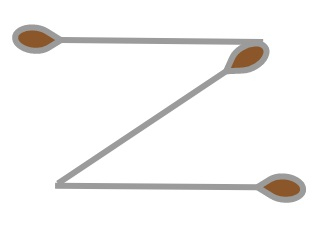From the figure, we observe that three matchsticks are required to make the letter Z.

Therefore, the required pattern is $3n$.

(c) A pattern of letter $\mathrm{U}$ as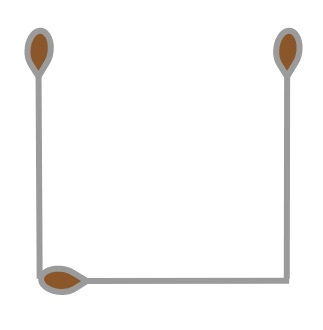From the figure, we observe that three matchsticks are required to make the letter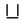.

Therefore, the required pattern is $3n$.

(d) A pattern of letter $\mathrm{V}$ as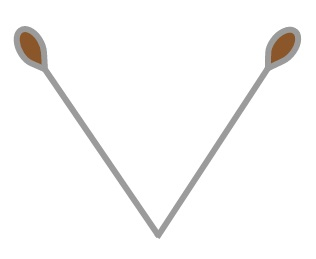From the figure, we observe that two matchsticks are required to make the letter V.

Therefore, the required pattern is $2n$.

(e) A pattern of letter $\mathrm{E}$ as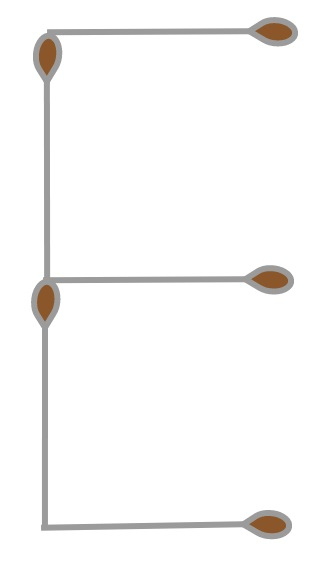From the figure, we observe that five matchsticks are required to make the letter E.

Therefore, the required pattern is $5n$.

(f) A pattern of letter $S$ as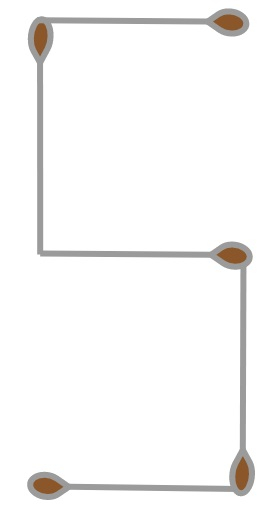From the figure, we observe that five matchsticks are required to make the letter.

Therefore, the required pattern is $5n$.

(g) A pattern of letter A as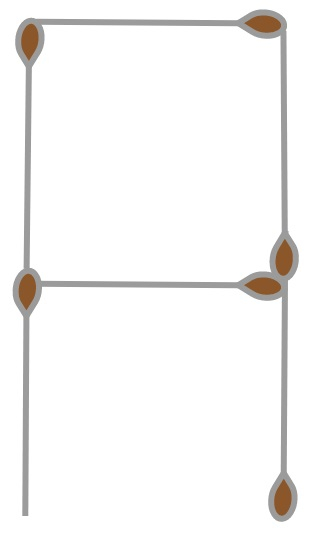From the figure, we observe that six matchsticks are required to make the letter.

Therefore, the required pattern is $6n$.